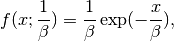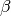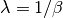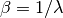# numpy.random.mtrand.RandomState.exponential¶

RandomState.exponential(scale=1.0, size=None)

Exponential distribution.

Its probability density function isfor x > 0 and 0 elsewhere.is the scale parameter, which is the inverse of the rate parameter. The rate parameter is an alternative, widely used parameterization of the exponential distribution [R149].

The exponential distribution is a continuous analogue of the geometric distribution. It describes many common situations, such as the size of raindrops measured over many rainstorms [R147], or the time between page requests to Wikipedia [R148].

Parameters : scale : float The scale parameter,. size : tuple of ints Number of samples to draw. The output is shaped according to size.

References

 [R147] (1, 2) Peyton Z. Peebles Jr., “Probability, Random Variables and Random Signal Principles”, 4th ed, 2001, p. 57.
 [R148] (1, 2) “Poisson Process”, Wikipedia, http://en.wikipedia.org/wiki/Poisson_process
 [R149] (1, 2) “Exponential Distribution, Wikipedia, http://en.wikipedia.org/wiki/Exponential_distribution